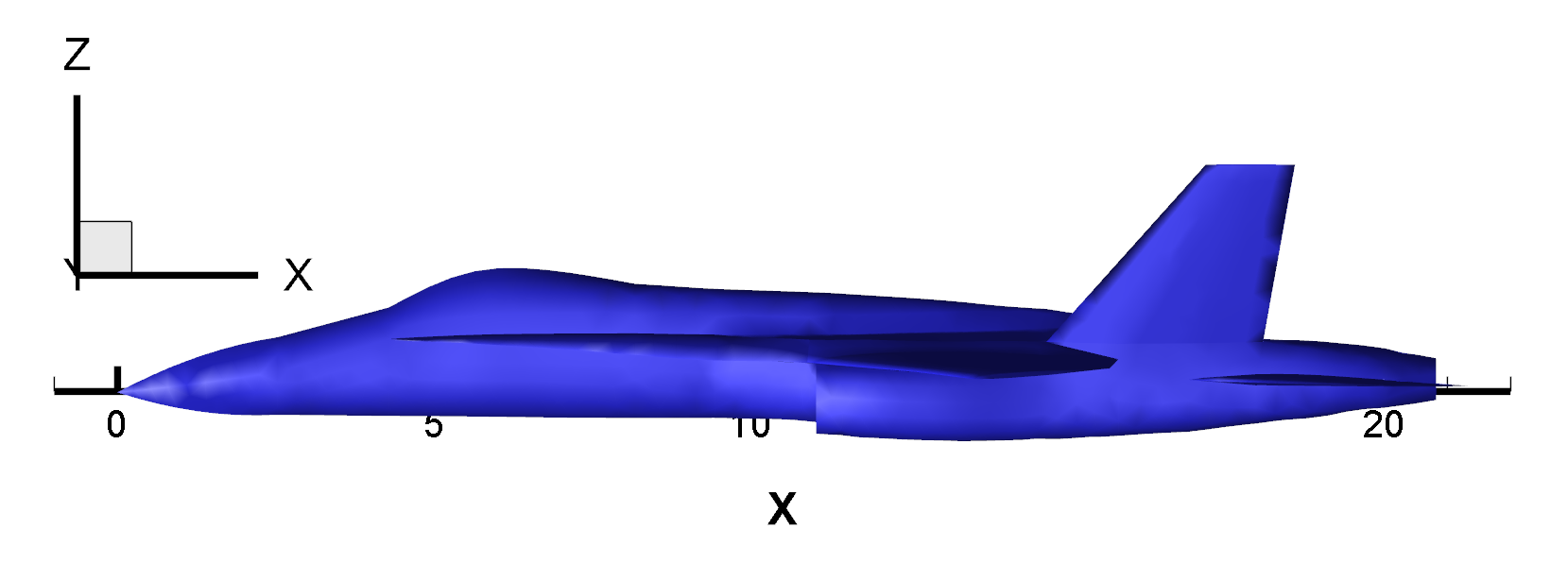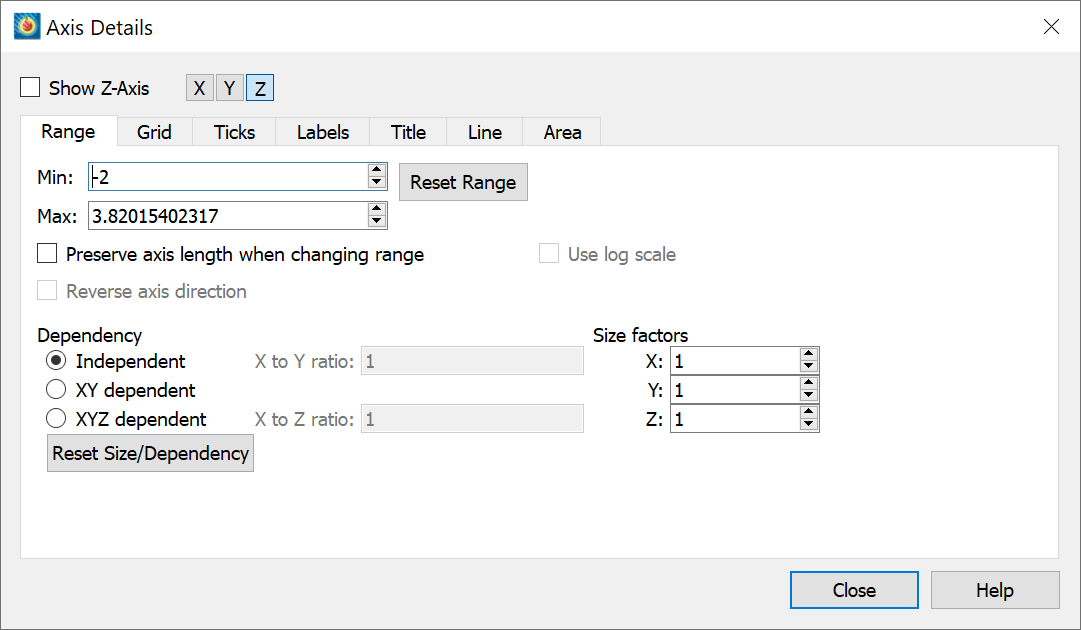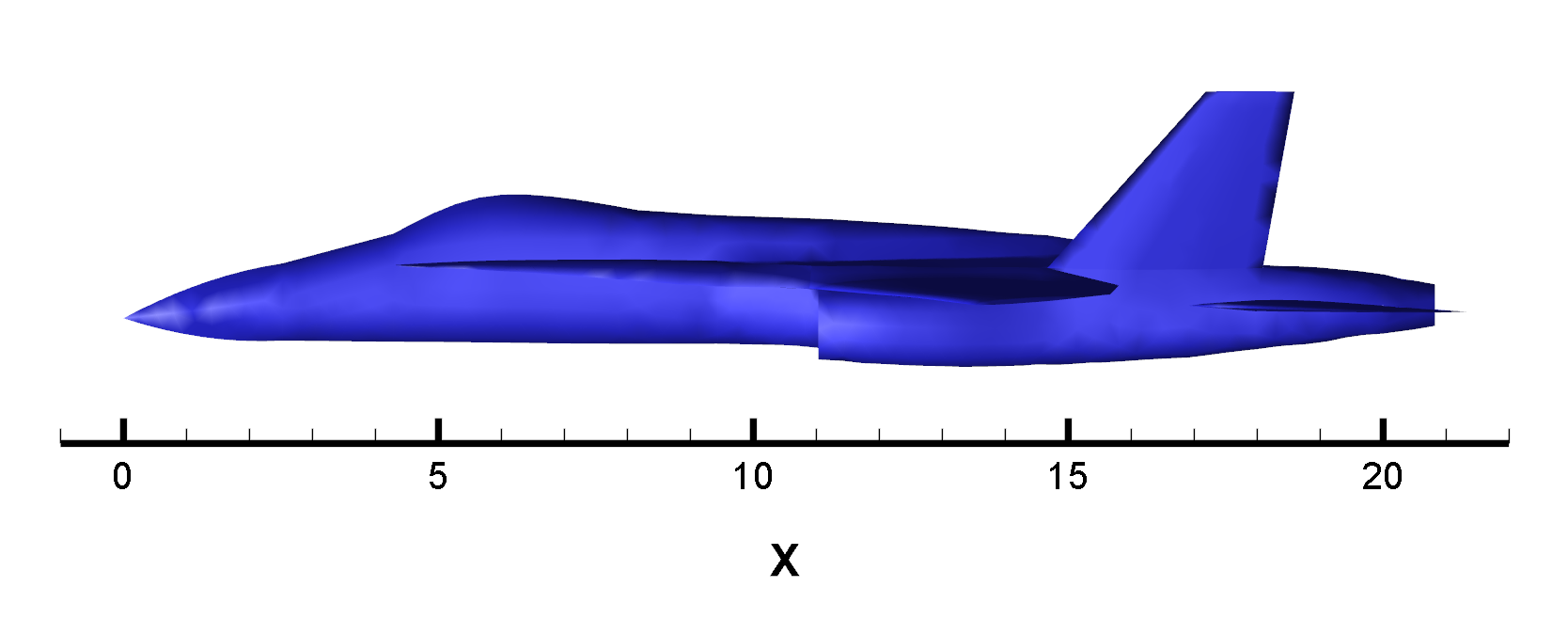# Offsetting an Axis in 3D Cartesian Mode

Problem:
I am displaying one axis in my 3D plot, but my data interferes with it (see Figure 1). How do I offset my axis away from my plot?Figure 1

Solution:
Change the minimum value of the vertical axis range in that view. In the case of Figure 1, the Z axis range minimum needs to be decreased.

For this example,
1) Go to Plot>Axis… and select the Z axis and make sure you are on the Range tab.
2) Adjust the range to an appropriate value.* In this case, decreasing from 0 to -2 works. See Figure 2.Figure 2

Now, the horizontal axis should be readable and sufficiently offset from the displayed data. See the final product in Figure 3.Figure 3

Note:
*  If the different axes are not in Independent mode and if Preserve axis length when changing range is toggled on, the perceived size of your plot may change when you adjust the Z range values. See Figure 2 for the settings used when creating the plot in Figure 3.